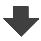Search:

# Chemical thermodynamics

## Enthalpy of formation

### Exercise 1

Calculate the standard enthalpy variation of the following reaction consulting the →   Table of formation enthalpies : $NH_3(g)$ $+$ $HCl(g)$ $\longrightarrow$ $NH_4Cl(s)$ (o = standard means that the thermodynamic parameters are to be calculated in stoichiometric proportion, that means that each species must have the same number of moles as given by the equation and that the reaction has to be complete)

$\Delta H^o$ $=$ $\Delta H_f(NH_4Cl(s))$ $-$ $(\Delta H_f(NH_3(g))$ $+$ $\Delta H_f(HCl(g)))$ $=$ $-101.16\;kJ$